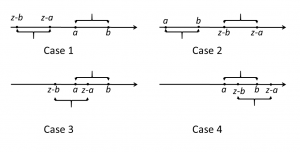1
May 18

## Sampling from uniform distribution - example of convolution

For the intuition behind the uniform distribution see this post. More formally, let us fix an interval$[a,b]$. We say that a random variable$X$ is uniformly distributed on$[a,b]$ if any value in this segment is equally likely and any value outside is impossible. The "equally likely" part gives a constant density value in the segment and the "impossible" part gives zero density outside the segment. Thus the density is of the form:$f_X(x)=\frac{1}{b-a}\left\{\begin{array}{ll}1, &x\in[a,b]\\0, &\text{otherwise}\end{array}\right.=\frac{1}{b-a}1_{[a,b]}(x).$

This is a step function divided by$b-a$ to make the total area under the graph equal to one. It is easy to find the distribution function of$f_X$ by considering three ranges of$x$:$F_X(x)=\left\{\begin{array}{ll}0,&x

## What is the density of a sum?

Suppose we have a sample of two independent observations$X,Y$ from the same uniform distribution. This means that they have the same density:$f_X(x)=f_Y(x)=\frac{1}{b-a}1_{[a,b]}(x).$

By independence we can apply the convolution formula (see equation (5)). The sum$X+Y$ has the density

(1)$f_{X+Y}(z)=\int_Rf_X(x)f_Y(z-x)dx=\frac{1}{(b-a)^2}\int 1_{[a,b]}(x)1_{[a,b]}(z-x)dx.$

Here$1_{[a,b]}(x)$ is different from zero only for

(2)$x\in[a,b]$.

Similarly,$1_{[a,b]}(z-x)$ is different from zero only for$z-x\in[a,b]$ or, equivalently, only for

(3)$x\in[z-b,z-a]$.

(2) and (3) show that the integrand in (1) is different from zero only for$x\in[z-b,z-a]\cap[a,b].$ So (1) becomes

(4)$f_{X+Y}(z)=\frac{1}{(b-a)^2}\int_{[z-b,z-a]\cap[a,b]}dx.$

Next we consider several cases depending on the relationship between the segments$[z-b,z-a],\ [a,b].$Figure 1. Relationship between segments

a) The two segments do not intersect:$z-a or$z-b>b$ (see Cases 1 and 2 in Figure 1). Equivalently, either$z<2a$ or$z>2b$. In this case the integral in (4) is zero.

b)$a\le z-a\le b$ or, equivalently,$2a\le z\le a+b$, see Case 3 in Figure 1. In this case the integral in (4) is equal to the length of the intersection:$z-2a$.

c) Finally,$a\le z-b\le b$ or, equivalently,$a+b\le z\le 2b$ (Case 4). Again, the integral in (4) equals the length of the intersection:$2b-z$.

Summarizing,

(5)$f_{X+Y}(z)=\frac{1}{(b-a)^2}\left\{\begin{array}{ll}0,&z<2a\ \text{or}\ z>2b;\\ z-2a,&2a\le z\le a+b;\\2b-z,&a+b\le z\le 2b.\end{array}\right.$Figure 2. Density of a sum of two observations

This is a triangular distribution, shown in Figure 2 from Mathematica with a=1, b=2. Note that it is smoother than the density of the uniform distribution: the density in Figure 2 is continuous and doesn't have jumps.Figure 3. Density of a sum of three observations

The density of the sum of three independent observations$X,Y,Z$ from the same uniform distribution is even smoother, see Figure 3. This is a general fact: as the number of independent observations$X_1,...,X_n$ (from the same population) increases, the density of the sum$X_1+...+X_n$ more and more reminds that of the normal distribution.

It is convenient to denote$U[a,b]$ the uniform distribution on the segment$[a,b]$ and$T[a,b]$ the triangular distribution defined in (5). We have proved that if$X,Y$ are independent and distributed as$U[a,b]$, then their sum is distributed as$T[a,b]$.